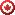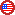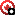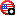Great Lakes Surf RadarYou Can Surf the Lakes!
 Home Old news MySpots Faves Writeups Contact Registration Login 2023-02-06, 03:01

[2022-10-21] Site update: Sheboygan Surf Cam Reinstated beach cam at Sheboygan, Lake Michigan

Plan your surfing sessions on the Great Lakes with surfradar.info! See summary of surf conditions and wave forecast for the Great Lakes for the USA and Canada. Drill down to the current buoy data and web cams for select spots. Best forecasts for surfers, bodyboarders, kiters, fishermen, yachtsmen and boaters on lake Ontario, lake Erie, lake Michigan, lake Huron and lake Superior. The Great Lakes surf forecast is refreshed every 3 hours from Great Lakes Coastal Forecasting System (GLCFS). Normally, the fun begins with reported waves of 3 ft (0.91 m), but still, please double check with the nearest buoy or surf-cam and read the Disclaimer. Currently we have surfcams at dashboards for Port Stanley ON, Sauble Beach ON by Sauble Trading Post, Grand Bend ON, Kincardine ON, Fifty Point ON (near Hamilton), Toronto ON, Rochester NY, Chicago IL, Holland MI (Grand Haven is nearby). There are also weather cams (surf line is not seen): Erie PA, Mentor, OH.
"You can't surf the Great Lakes" :-) videos. Read this Surfer mag article on essence of Surfing the Great Lakes.
Latest Great Lakes surf nowcast from GLERL-GLCFS (next-gen FVCOM), with spots by surfradar.info Point onorto see spot's name, click it for spot's forecast and details..anddenote spots with surfcams.
You can also chose your favorite spots in MySpots Area Selector.

The summary forecast table below is generated at 02/06/2023 01:50 EST (data 02/05/2023 21:40 EST). See individual spot pages and the latest GLERL's maps for the most current data.
The chart shows when to expect maximum wave Significant Height at given Spots. The 1st number is expected maximum wave Significant Height in feet, then hour or begin-end hours.
"~" means surf drop between peaks; "–" designates consistent surf expected between start and end time.
CountryLakeSpotSun Feb 05Mon Feb 06Tue Feb 07Wed Feb 08Thu Feb 09Fri Feb 10USAErieAshtabula0 at 21:00–23:000 at 01:00–23:000 at 02:00–20:000 at 02:00–20:004 at 14:005 at 02:00USAErieBuffalo0 at 21:00–23:000 at 01:00–23:000 at 02:00–20:000 at 02:00–20:002 at 14:003 at 14:00USAMichiganChicago2 at 21:00–23:005 at 23:006 at 02:00–05:003 at 02:00~20:004 at 02:004 at 14:00USAErieCleveland0 at 21:00–23:000 at 01:00–23:000 at 02:00–20:000 at 02:00–20:004 at 14:003 at 02:00CanadaOntarioCobourg4 at 22:003 at 01:007 at 17:003 at 08:003 at 14:002 at 08:00–14:00USASuperiorDuluth1 at 21:00–23:001 at 01:00–23:001 at 02:00–20:001 at 02:00–20:001 at 02:00–20:001 at 02:00–14:00USAMichiganEmpire3 at 21:00–23:004 at 23:006 at 05:003 at 02:00–14:003 at 14:004 at 02:00~14:00USAEriePresquile SP0 at 21:00–23:001 at 20:00–23:001 at 02:001 at 14:00–20:006 at 20:006 at 02:00CanadaOntarioFifty Point1 at 21:00–23:002 at 04:00–11:003 at 11:002 at 02:003 at 20:002 at 02:00–14:00USAMichiganFrankfort4 at 21:004 at 23:007 at 08:003 at 02:00–20:005 at 20:005 at 08:00USAErieFreeport Beach1 at 22:000 at 01:00–23:000 at 02:00–20:001 at 14:00–20:003 at 08:006 at 02:00CanadaHuronGrand Bend2 at 23:002 at 01:00~08:000 at 02:00–20:001 at 14:00–20:003 at 20:004 at 02:00USAMichiganGrand Haven4 at 21:00–23:003 at 01:00–03:009 at 08:004 at 02:007 at 14:005 at 02:00–08:00USASuperiorGrand Marais5 at 23:003 at 01:00–02:008 at 17:001 at 14:00–20:005 at 20:007 at 08:00CanadaOntarioHamilton1 at 21:00–23:001 at 01:00–23:004 at 14:002 at 02:003 at 14:00–20:002 at 02:00USAMichiganHolland5 at 21:004 at 01:008 at 08:004 at 02:007 at 14:006 at 02:00USAMichiganIndiana Dunes3 at 21:00–23:004 at 23:005 at 02:00–08:003 at 02:00~20:004 at 14:00~20:005 at 14:00USASuperiorKeweenaw North2 at 21:00–23:003 at 23:008 at 14:002 at 08:00~20:006 at 20:007 at 02:00CanadaHuronKincardine5 at 23:005 at 01:00–04:006 at 17:004 at 02:004 at 20:004 at 02:00–08:00CanadaErieLeamington0 at 21:00–23:000 at 01:00–23:000 at 02:00–20:000 at 02:00–20:000 at 02:00–20:000 at 02:00–14:00CanadaHuronMeaford2 at 22:004 at 03:00–06:005 at 11:00–14:002 at 08:004 at 20:003 at 02:00–14:00USAErieMentor0 at 21:00–23:000 at 01:00–23:000 at 02:00–20:000 at 02:00–20:007 at 20:005 at 02:00USAMichiganMilwaukee1 at 21:00–23:006 at 23:006 at 02:00~11:004 at 02:00–20:006 at 08:003 at 14:00USAMichiganOgden Dunes3 at 21:00–23:005 at 23:006 at 02:00–08:003 at 02:00~20:003 at 02:003 at 02:00–08:00CanadaOntarioOshawa0 at 21:00–23:001 at 01:00~23:001 at 02:002 at 14:001 at 02:00–08:001 at 08:00–14:00CanadaOntarioPickering2 at 21:00–23:002 at 07:00–08:007 at 14:003 at 02:004 at 20:002 at 02:00–08:00CanadaEriePoint Pelee0 at 21:00–23:000 at 01:00–23:000 at 02:00–20:000 at 02:00–20:000 at 02:00–20:001 at 08:00–14:00CanadaEriePoint Pelee Tip0 at 21:00–23:000 at 01:00–23:000 at 02:00–20:000 at 02:00–20:000 at 02:00–20:000 at 02:00–14:00CanadaEriePort Colborne5 at 21:00–22:003 at 01:00–04:008 at 20:007 at 02:003 at 14:00–20:003 at 02:00USAHuronPort Huron0 at 21:00–23:000 at 01:00–23:000 at 02:00–20:000 at 02:00–20:001 at 02:00–20:001 at 02:00–14:00CanadaEriePort Stanley0 at 21:00–23:000 at 01:00–23:000 at 02:00–20:000 at 02:00–20:008 at 20:006 at 02:00CanadaOntarioPresqu'ile Point5 at 21:00–22:003 at 01:00–04:008 at 20:007 at 02:003 at 14:00–20:003 at 02:00USAOntarioRochester1 at 21:00–23:004 at 08:00–11:003 at 11:00~20:004 at 02:002 at 14:00–20:003 at 08:00CanadaOntarioSandbanks PP5 at 21:00–22:004 at 01:007 at 11:00~20:008 at 02:002 at 02:00–08:004 at 02:00USAErieSandusky1 at 21:00–23:001 at 01:00~23:001 at 02:00~20:001 at 02:00~20:004 at 08:00–20:003 at 02:00CanadaHuronSarnia0 at 21:00–23:000 at 01:00–23:000 at 02:00–20:000 at 02:00–20:000 at 02:00–20:000 at 02:00–14:00CanadaHuronSauble Beach4 at 21:003 at 03:000 at 02:00–20:005 at 02:002 at 02:00~20:002 at 02:00–14:00USAOntarioSelkirk Shores3 at 21:00–23:003 at 01:00–11:005 at 14:00–20:008 at 02:002 at 02:00~20:003 at 02:00–14:00CanadaErieSelkirk PP1 at 21:00–23:002 at 04:00~23:006 at 14:002 at 08:00–14:000 at 02:00–20:000 at 02:00–14:00USAMichiganSheboygan1 at 21:00–23:002 at 23:004 at 17:00–20:004 at 02:00~20:004 at 02:001 at 14:00USAOntarioSouthwick Beach3 at 21:00–23:003 at 01:00–08:006 at 14:00–20:009 at 02:002 at 02:00–20:004 at 08:00USASuperiorStoney Point1 at 21:00–23:001 at 01:00–23:002 at 08:00–11:001 at 02:00–20:002 at 14:00–20:002 at 02:00CanadaOntarioToronto2 at 21:00–23:002 at 08:006 at 14:00–17:003 at 02:004 at 20:002 at 02:00–08:00CanadaHuronWasaga Beach2 at 21:00–23:003 at 01:00–07:006 at 14:005 at 02:002 at 02:00~20:003 at 02:00USAMichiganWhiting2 at 23:005 at 23:005 at 02:00–05:002 at 02:00–20:003 at 02:001 at 02:00–14:00CADUSD